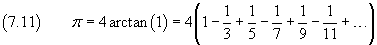# Calculating an approximate value of PI, another method

I was having a look at a method of calculating an approximate value of PI without resorting on random numbers. Instead, I wanted something using the principles of limits and series.

We are not going to find a lot of digits of PI. We are just calculating an approximate value of PI with a few but correct digits. Something faster and more precise than what Monte Carlo Method can offer.

### The principle

We know from math that PI = 2 * lim (arctg(x), x -> +oo). This just means that if we take the arctangent of a very, very big number and we multiply the result by two, we get something quite close to the value of PI.

But, since we are going to calculate it by ourselves, we are not going to use the function ATN provided by BASIC 2.0. Instead, we want to use simple computations.

Starting from the Taylor/Mc Laurin series for arctangent(x), it can be proven that:So, we can simply use this formula to get an approximation of PI. We need to create a loop that sums up those endless terms and shows continuously the updated result on screen. The user will stop the program once he gets a satisfying approximation or when digits stop changing on screen (if he happens to be patient enough).

Look at the terms on parenthesis. Odd terms are positive, even terms are negative. How can we handle this in the code? One solution may be to use a variable equal to 1, and then reversing its sign each time. For instance, if variable is S, first of all we put S = 1, then we reverse its sign each time with the statement S = -S.

At this point, calculating each term is just a matter of dividing 1 by a counter. This counter (D in the code) will be assigned a 1 as a starting value, then it will be incremented by 2 on each loop. So, we get the divisors 1, 3, 5, 7, 9, 11… like on the terms in the above formula.

We can sum each term that we compute on a loop to the variable P, again and again. Then, P*4 will contain the approximate value of PI.

On the program, variable P is initialized to 1. That’s because we already take for computed the first term of the series, which is simply 1.

### The code

The code is very short – just two lines.